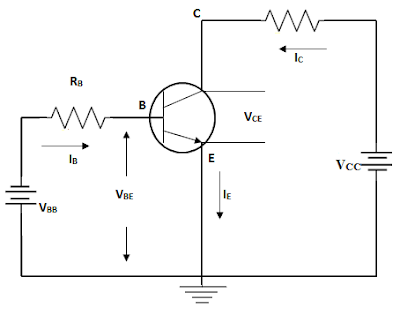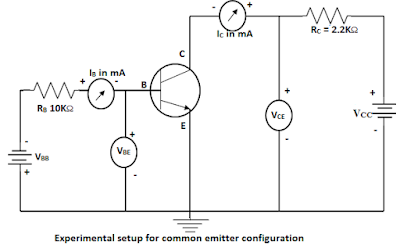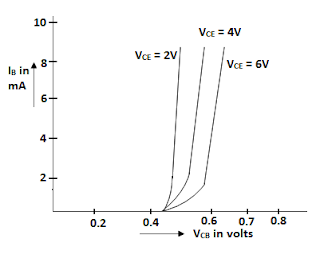# Common Emitter Configuration

Consider an n-p-n transistor in common emitter configuration. In this configuration the base current IB is the input current and collector current IC is the output current.We know  IC = IC + IB
and IC = αIE + ICBO
= (IC + IB) + ICBO or
IC (1-α) = αIB + ICBO
IC = [α/(1-α)]IB + ICBO/(1-α)

# Common Emitter Configuration

#### Current Amplification Factor

The ratio of the collector current to the base current is called dc forward current transfer ratio or the dc current gain. It is designated by βdc. It is said to be the dc beta.

Common Emitter dc current gain βdc = IC/IB
​Where IC and IB are collector and base current of a particular operating point in the linear region. As the value of base current is in microamperes and low, the value of βdc lies in the range of 10 to 500 depending on the type of the transistor.

Common emitter ac current gain βdc is defined as the ratio of small change in collector current ΔIB at a constant collector to emitter voltage VCE.

βdc = ΔIC/ΔIE    AT  VCE = constant​

#### Relation between αdc and βdc

We know the                I E = IC + IB  ----> i
Dividing by IC
IE/IC = 1+(IB/IC)
1/α = 1 + (1/β)   =  (β+1) / β                  [ ∴ α = IC / IE and β = IC/IB]
α = β / (β+1)  ------>  ii
Cross multiplying the above equation, we have,
α(β+1) =β
αβ+α =β
α = β – αβ  = β (1 – α)
β = α / (α+1)  -------> iiii

Equation (ii) --->
α = β / (β+1)
1-α = 1 – [β / (β+1)]  ------>iv
From equation (iii), it is seen that as α approaches unity, β approaches infinity.

That is the current gain of transistor in common emitter configuration is very high. It is because of this reason that transistors are used in common emitter configuration.

We know that, IC = [αIB / (1-α)] + ICBO / (1-α)   ----->v
Since,  β+1 = 1 / (1-α) and  1-α= 1 / (β+1), We have
IC = βIB + (β+1) ICBO  ------->vi

​The term “(β+1)ICBO” is the reverse leakage current in common emitter configuration. It is denoted by ICEO.
ICEO = (β+1)ICBO   ------>vii

​Since β is much greater than I, ICEO>ICBO.
Substituting the value of ICEO in equation vi, we get
IC = βIB + ICEO    ------->viii

Since, β = α / (α+1), we can write
ICEO = [α / (1-α)] ICBO  ------->ix

ICEO = ICEO / (1-α )
• The leakage current in common emitter configuration is larger than that of common base configuration. ICE is the collector current which flows when the base emitter circuit is left open and collector base junction is reverse biased. It is in the same direction as normal collector current flows through the transistor and is temperature sensitive.

## Characteristic of Common Emitter ConfigurationThe figure above shows that the experimental setup for determining the static characteristic of an n-p-n transistor used in a common emitter configuration circuit. Two variable dc regulated power supplies VBB and VCC are connected to base and collector terminals of a transistor.
• A micro ammeter and a voltmeter are connected to measure the base current IB and VBE, and a milli ammeter and a voltmeter are connected to measure IC and VCE in the circuit.

#### Input Characteristic (VBE vs IB) VCE= Constant

The input characteristic curves are obtained by plotting base emitter voltage VBE vs base current IB keeping VCE constant. The characteristic curves are plotted for various values of collector to emitter voltage VCE.From the input characteristic we observe the following important points:
• There exist a threshold, cut in voltage Vγ below which the base current IB is very small. The value of cut in voltage is 0.5 V for Si and 0.1V for GE transistors.
• After the cut in voltage the base current IB increases rapidly with small increase in base-emitter voltage VBE. However, it may be noted that the value of base current does not increases as rapidly as that of the input characteristic of a common base configuration. It means that dynamic input resistance is small in common emitter configuration but it is little higher as compared to the CB configuration.
• For a fixed value of VBE, IB increases as VCE is decreased. A large value of VCE results in a larger reverse bias at collector base PN junction. This increases the depletion region and reduces the effective width if the base, which in turn reduces the base current.
• Dynamic or ac input resistance can be determined from the input characteristics curve. It is defined as the ration of a small change in the base to emitter voltage to the resulting change in the base current at constant to emitter voltage.
Input Resistance RO = [ΔVBE/ΔIB]      at VCE = constant

The value of Rin is typically 1KΩ, but can range from 600Ω to 4KΩ.

#### Output Characteristics (VCE vs IC) at IB=Constant• The output characteristic of the common emitter configuration curves are obtained by plotting VCE vs. IC for different values of IB. The collector current varies with VCE for values between 0V and 1V. The collector current varies with VCE for values between 0V and 1V. After this collector current IC becomes almost constant and reaches the saturation values. The transistors are operated in the region above knee voltage. This region is called active region. The experiment is repeated for different values of IB.
• The output characteristics curves may be divided into three regions. They are
1. Saturation region
2. cut-off region
3. Active region
• In the above figure the active region is the area to the right of the ordinate VCE = a few tenths of a voltage and above of IB = 0.
• Ideally, when VCE exceeds 0.7V, the base collector junction becomes reverse biased and the transistor goes into the active or linear region of its operation. Once the base collector junction is reverse biased IC levels off and remains almost constant for a given value of IB as VCE continues to increase. Actually IC increases very slight as VCE increases due to widening of the base collector depletion region. This phenomenon is called a nearly effect.
• When the base current IB is zero, a small collector current exists. This is called leakage current. However, for all practical purposes, the collector current is zero, when the base current is zero. Under this condition the transistor is said to be cut-off. The small collector current is called the collector cut-off current.
• When VCE reaches a sufficiently high voltage, the reverse biased collector junction goes into breakdown and therefore collector current increases rapidly. If VCE is greater than 40 V, the collector diode breaks down and normal transistor action is lost. The transistor is not intended to operate in the breakdown region. This effect is commonly known as punch through effect.
• From the output characteristics, the dynamic output resistance can be determined. It is given by

Dynamic output resistance Rout = [ΔVCE/ΔIC]      at IB = constant
• The reciprocal of the slope of the output characteristic in the region gives the output resistance. The value of Rout ranges from 10KΩ to 50 KΩ.
• The output characteristic may be used to determine the small signal common emitter current gain or ac beta (βac) of a transistor. This can be done by selecting the two points M and N on the characteristic and note the corresponding values of ΔIE and ΔIB.
βdc = ΔIC / ΔIE  = 10mA / 40mA  =250.0 CommentsComments